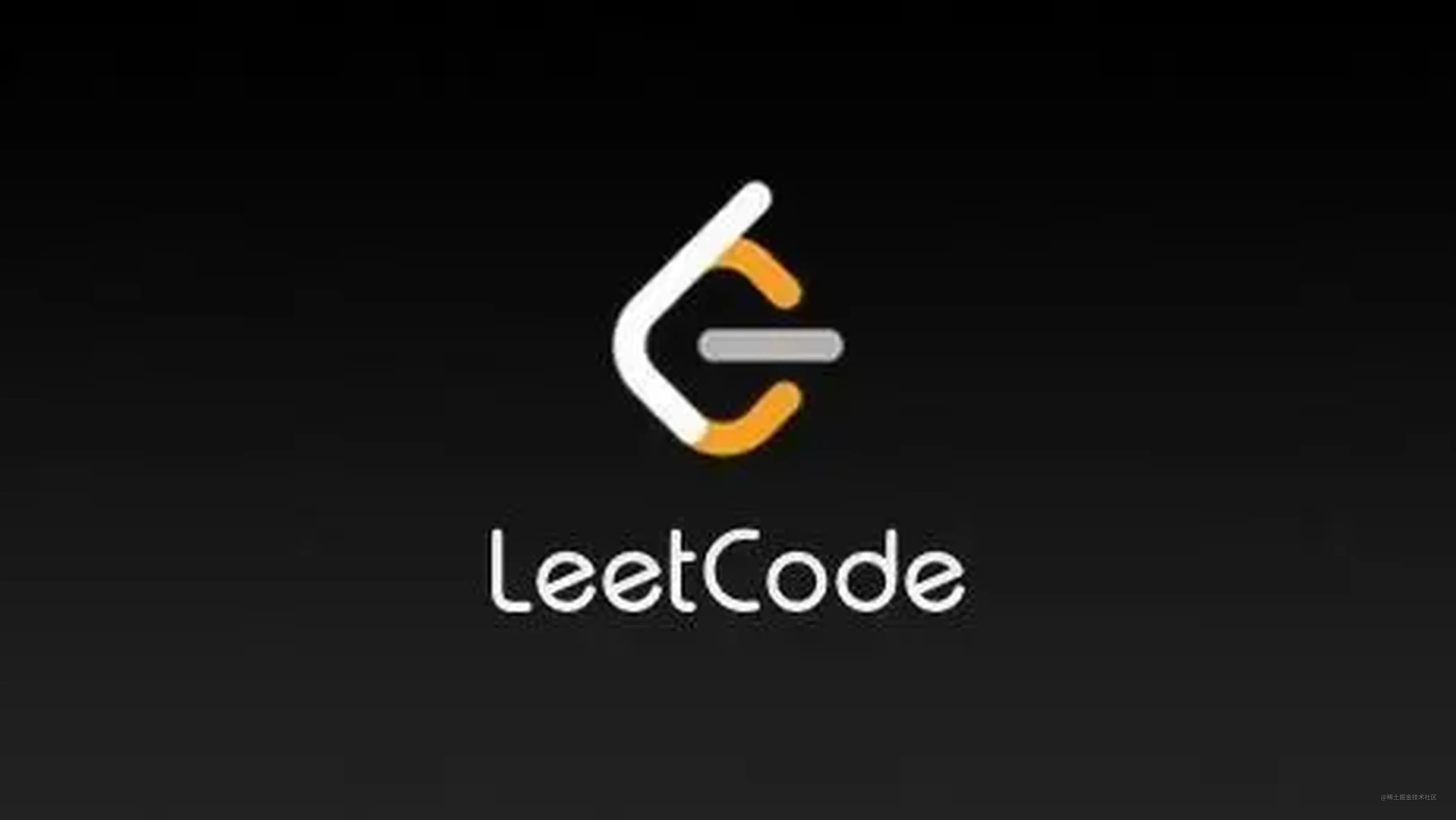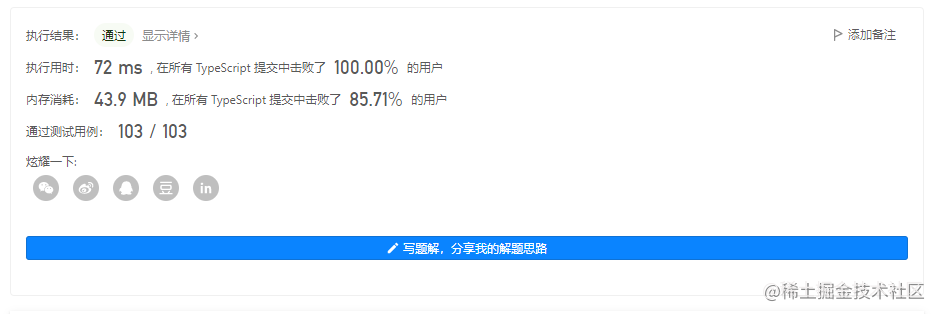# leetcode刷题记录-1403. 非递增顺序的最小子序列## 每日一题

• 给你一个数组 nums，请你从中抽取一个子序列，满足该子序列的元素之和 严格 大于未包含在该子序列中的各元素之和。

• 如果存在多个解决方案，只需返回 长度最小 的子序列。如果仍然有多个解决方案，则返回 元素之和最大 的子序列。

• 与子数组不同的地方在于，「数组的子序列」不强调元素在原数组中的连续性，也就是说，它可以通过从数组中分离一些（也可能不分离）元素得到。

• 注意，题目数据保证满足所有约束条件的解决方案是 唯一 的。同时，返回的答案应当按 非递增顺序 排列。

``````输入：nums = [4,3,10,9,8]

``````输入：nums = [4,4,7,6,7]

``````输入：nums = 

• 1 <= nums.length <= 500
• 1 <= nums[i] <= 100

## 题解

### 排序+贪心

``````function minSubsequence(nums: number[]): number[] {
let sum = nums.reduce((a,b) => a+b)
let cur = 0
let n = nums.length - 1
nums = nums.sort((a,b)=>a-b)
const ans = new Array<number>()
while (cur <= sum) {
sum -= nums[n]
cur += nums[n]
ans.push(nums[n])
n--
}
return ans
};